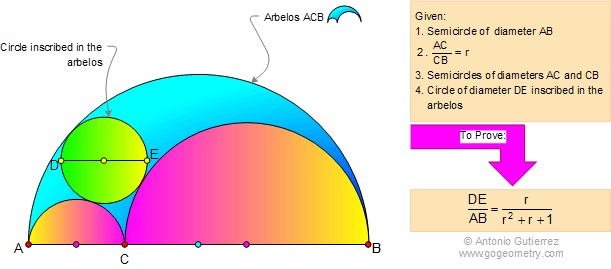# Geometry Problem 645: Archimedes' Book of Lemmas: Proposition 6, Arbelos

Let AB, the diameter of a semicircle, be divided at C so that $$\dfrac{AC}{CB}=r$$. If DE is the diameter of the circle inscribed in the arbelos ACB, then $$\dfrac{DE}{AB}=\dfrac{r}{r^2+r+1}$$.
"Arbelos" means shoemaker's knife in Greek.## Lemma 6 with Archimedes in the background as shown in 1584 book by Andre Thevet## Books of Lemmas Index

 Home | Search | Geometry | Problems | All Problems | Visual Index | 641-650 | Archimedes' Lemmas | Arbelos | Semicircle | Email | Post a comment or solution | by Antonio Gutierrez